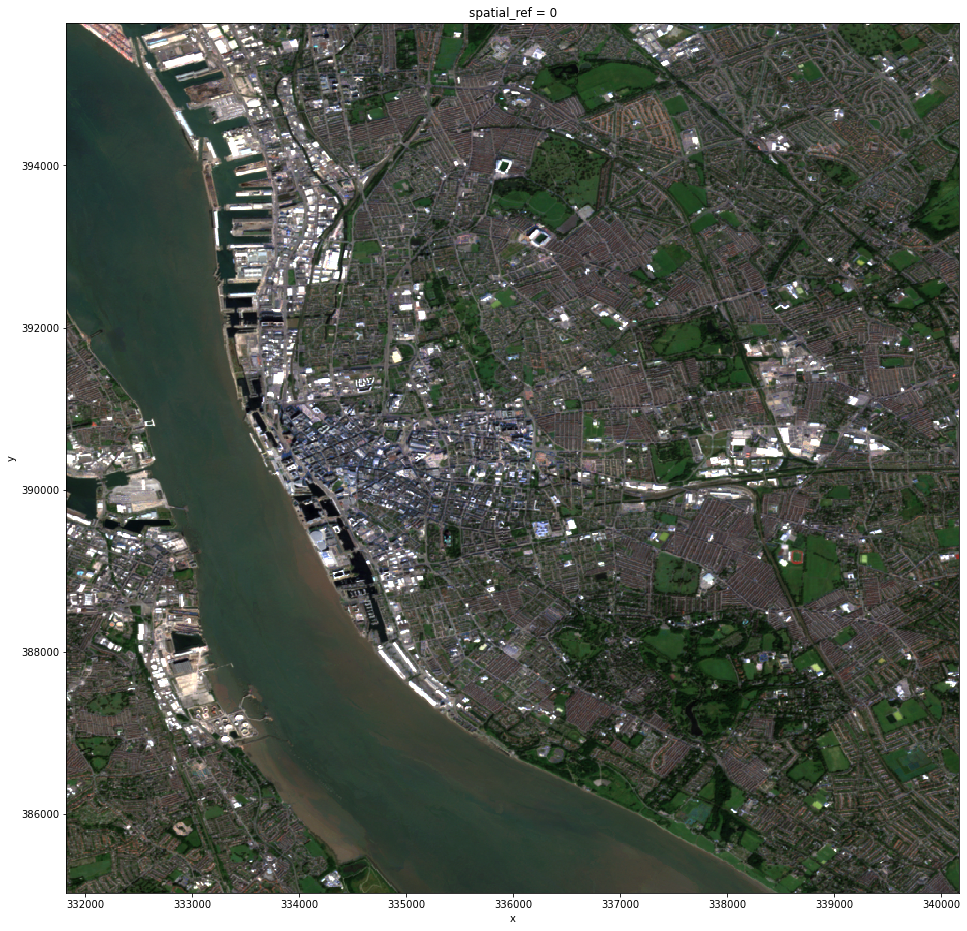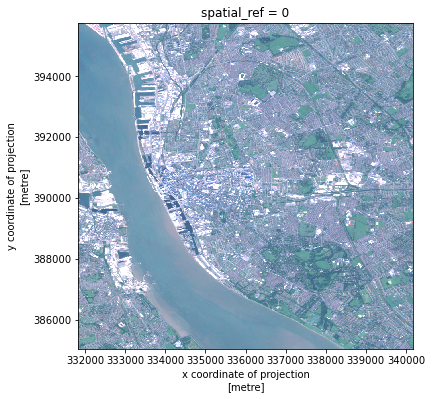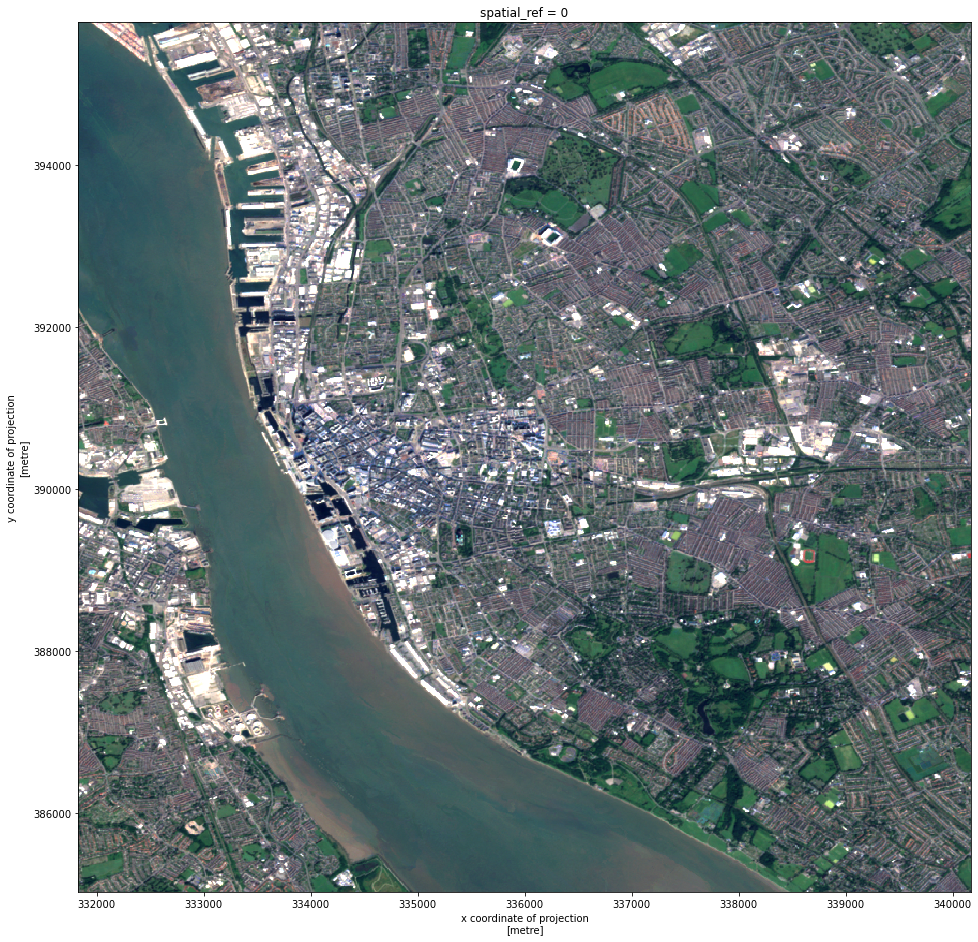# Chip making¶

This document shows how we flexibly generate a grid of pixel patches (or chips) and select those that fully fall within a given signature class. The result needs to fit in memory.

import os, time
import pandas
import geopandas
import tools
import xarray, rioxarray
# from geopandas_view import view
from shapely.geometry import box

from joblib import Parallel, delayed

tmp_dir = '/home/jovyan'
out_f_xys = f'{tmp_dir}/chip_xys_liv'
grid_dir = f'{tmp_dir}/grid'
joined_dir = f'{tmp_dir}/joined'

v0.1.0a5+8.ge953ff8

client = Client()
client

/opt/conda/lib/python3.9/site-packages/distributed/node.py:160: UserWarning: Port 8787 is already in use.
Perhaps you already have a cluster running?
Hosting the HTTP server on port 38187 instead
warnings.warn(


### Client

Client-138f2b0b-7d00-11ec-8fcb-5d66f227cdbe

 Connection method: Cluster object Cluster type: distributed.LocalCluster Dashboard: http://127.0.0.1:38187/status

### Cluster Info

• Signatures (simplified)

sigs = geopandas.read_file(
'/home/jovyan/data/spatial_signatures/signatures_combined_levels_simplified.gpkg'
)

/opt/conda/lib/python3.8/site-packages/geopandas/geodataframe.py:577: RuntimeWarning: Sequential read of iterator was interrupted. Resetting iterator. This can negatively impact the performance.
for feature in features_lst:

• Mosaic

r = rioxarray.open_rasterio(
'../urbangrammar_samba/ghs_composite_s2/GHS-composite-S2.vrt',
chunks={'x': 1024, 'y': 1024}
)


Liverpool for prototyping:

bb = box(331825, 385032, 340167, 395756)
# bb = box(321566, 468106, 365379, 437198)
liv = r.sel(
band=[1, 2, 3]
).rio.clip_box(
*bb.bounds
).compute()

def minmax(
a,
bottom=0,
top=255,
min_cut=0,
max_cut=99
):
from numpy import percentile
vals = a.to_series().values
min_bin = percentile(vals, min_cut)
max_bin = percentile(vals, max_cut)
a = xarray.where(a > max_bin, max_bin, a)
a = xarray.where(a < min_bin, min_bin, a)
a_std = (a - a.min()) / (a.max() - a.min())
a_scaled = a_std * (top - bottom) + bottom
return a_scaled.astype(int)

liv.groupby(
"band"
).map(
minmax
).plot.imshow(figsize=(16, 16));(liv / (1500 / 255)).astype(int).plot.imshow(figsize=(6, 6));

Clipping input data to the valid range for imshow with RGB data ([0..1] for floats or [0..255] for integers).bb = box(331825, 385032, 340167, 395756)
# bb = box(321566, 468106, 365379, 437198)
liv = r.sel(
band=[1, 2, 3]
).rio.clip_box(
*bb.bounds
).compute()

img = liv.values

for i, (l_min, l_max) in enumerate([(350, 1600), (500, 1600), (600, 1800)]):
img[i][img[i] > l_max] = l_max
img[i][img[i] < l_min] = l_min
a_std = (img[i] - l_min) / (l_max - l_min)
img[i] = a_std * 255

liv2 = liv.copy()
liv2.values = img
liv2.plot.imshow(figsize=(16, 16))

<matplotlib.image.AxesImage at 0x7fbc40780bb0>min_bin = 350
max_bin = 1500
a = xarray.where(a > max_bin, max_bin, a)
a = xarray.where(a < min_bin, min_bin, a)
a_std = (a - a.min()) / (a.max() - a.min())
a_scaled = a_std * (top - bottom) + bottom
return a_scaled.astype(int)


## Build the grid¶

The goal here is to have a flexible and performant method to generate a uniform grid of polygons (GeoDataFrame) that matches the layout of pixels in the mosaic.

### Small datasets¶

For small datasets, we can all pack it into a single shot:

%%time
liv_grid = tools.build_grid(
liv.coords['x'], liv.coords['y'], 6, crs=liv.rio.crs
)

CPU times: user 4.34 s, sys: 60.9 ms, total: 4.4 s
Wall time: 1.51 s


### Larger-than-RAM datasets¶

Datasets that do not fit in memory can be run through dask-geopandas, but it is worth splitting the job across different steps.

• [X] Turn coords into grid XYs as a dask.DataFrame w/ Point objects –> chip_pts

• [X] Turn chip_pts into pixel squares –> grid

• [ ] Join sigs to grid to transfer label and discard mixed-signature chips –> sig_chips

To select the number of chunks to write (npartitions), we can do a back-of-the-envelope calculation:

• The mosaic is 121,865 by 182,437 pixels (22,232,685,005) with four values per pixel

• 1500 partitions allocates +59m. ints per chunk (200Mb approx in memory)

## Deprecated¶

First we get the coordinates for the centroid of each chip written to disk:

%%time
! rm -rf $out_f_xys xys, chip_len = tools.coords2xys_parquet( out_f_xys, # Folder to dump chunks on disk 8, # No. of chunks liv.coords['x'], # X coords liv.coords['y'], # Y coords chip_res=10 # Chip size in pixels )  CPU times: user 15.3 s, sys: 270 ms, total: 15.6 s Wall time: 2.48 s  These are now in disk, so we can work with them within dask/-geopandas. ! du -h$out_f_xys

3.6G	/home/jovyan/chip_xys


Next step involves turning point coordinates into points. For now, we just express the computation:

%%time

def process_chunk(xy_pXgrid_p):
t0 = time.time()
xy_p, grid_p = xy_pXgrid_p
chip_len = abs(
)
xy_pts = geopandas.points_from_xy(
xys['X'], xys['Y']
)
grid = xy_pts.buffer(chip_len/2, cap_style=3)
geopandas.GeoDataFrame(
{'geometry': grid}, crs='EPSG:27700'
).to_parquet(grid_p)
t = time.time() - t0
msg = f'Execution of {grid_p.split("/")[-1]} completed in {t} seconds'
return msg

! rm -rf $grid_dir ! mkdir$grid_dir
items = [
(
f'{out_f_xys}/chunk_{i}.pq', f'{grid_dir}/chunk_{i}.pq'
) for i in range(len(os.listdir(out_f_xys)))
]

'''

CPU times: user 37.7 ms, sys: 107 ms, total: 145 ms

'\n_ = tools.dask_map_seq(process_chunk, items[:16], client)\nout = Parallel(n_jobs=16)(\n    delayed(process_chunk)(i) for i in items\n)\n'